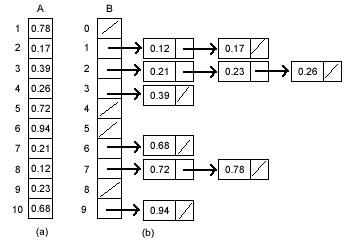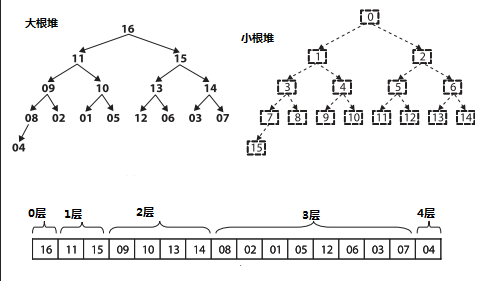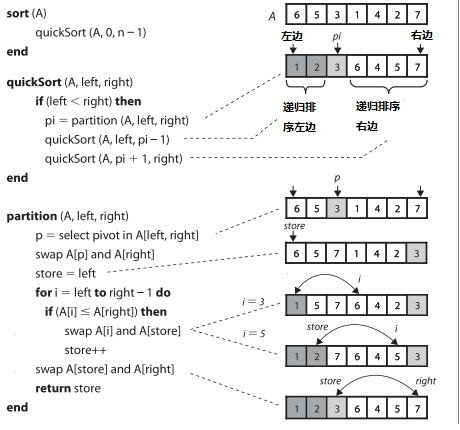# 算法之美：排序

1.全部为整数(我们学校的考试成绩没有小数)     2.没有负数(成绩最低为0)     3.有范围（0 ～ 100）

O(n)，换言之，计数排序只需要扫描待排序列一次就可以完成排序了。用这种排序轻松的解决了童鞋的问题。

。这种排序法就叫做计数排序。

```void countsort(int * arr, int n, int k)//排序arr中n个元素，范围是[0,K)
{
int i, idx = 0;
int *B = calloc(k, sizeof(int));
for(i = 0; i < n; i++)
B[arr[i]]++;
for(i = 0; i < k; i++)
while(B[i]-- > 0)
arr[idx++] = i;```
```     free(B);
}
```

```void countsort(BYTE *array, int length)
{
int t;
int i, z = 0;
BYTE min,max;
int *count;
min = max = array;
for(i=0; i<length; i++)
{
if(array[i] < min)
min = array[i];
else if(array[i] > max)
max = array[i];
}
count = (int*)malloc((max-min+1)*sizeof(int));
for(i=0; i<max-min+1; i++)
count[i] = 0;
for(i = 0; i < length; i++)
count[array[i]-min]++;

for(t = 0; t <= 255; t++)
for(i = 0; i < count[t-min]; i++)
array[z++] = (BYTE)t;
free(count);
}```

1.输入的数据要均匀的分布在一个给定的范围内。2.桶必须是有序的。```//伪代码
const int nBuckets = (MAX / 10) + 1;

Bucket bucket [nBuckets];

for (i = 0; i < n; i++)
bucket [A[i] / 10].insert (A[i]);

for (i = 0; i < nBuckets; i++)
bucket [i].sort ();

for (i = 0; i < nBuckets; i++)
cout << bucket [i];```

```#include <iostream.h>

class element
{
public:
int value;
element *next;
element()
{
value=NULL;
next=NULL;
}
};

class bucket
{
public:
element *firstElement;
bucket()
{
firstElement = NULL;
}
};

void main()
{
int lowend=0;
int highend=100;
int interval=10;
const int noBuckets=(highend-lowend)/interval;
bucket *buckets=new bucket[noBuckets];
bucket *temp;

for(int a=0;a<noBuckets;a++)
{
temp=new bucket;
buckets[a]=*temp;
}
int array[]={12,2,22,33,44,55,66,77,85,87,81,83,89,82,88,86,84,88,99};

for(int j=0;j<19;j++)
{
cout<<array[j]<<endl;
element *temp,*pre;
temp=buckets[array[j]/interval].firstElement;
if(temp==NULL)
{
temp=new element;
buckets[array[j]/interval].firstElement=temp;
temp->value=array[j];
}
else
{
pre=NULL;
while(temp!=NULL)
{
if(temp->value>array[j])
break;
pre=temp;
temp=temp->next;
}
if(temp->value>array[j])
{
if(pre==NULL)
{
element *firstNode;
firstNode=new element();
firstNode->value=array[j];
firstNode->next=temp;
buckets[array[j]/interval].firstElement=firstNode;
}
else
{
element *firstNode;
firstNode=new element();
firstNode->value=array[j];
firstNode->next=temp;
pre->next=firstNode;
}
}
else
{
temp=new element;
pre->next=temp;
temp->value=array[j];
}

}
}
for(int jk=0;jk<10;jk++)
{
element *temp;
temp= buckets[jk].firstElement;
while(temp!=NULL)
{
cout<<"*"<<temp->value<<endl;
temp=temp->next;
}
}

}
`````` #define MAX_HEAP_LEN 100
static int heap[MAX_HEAP_LEN];
static int heap_size = 0;              // 堆的大小

static void swap(int* a, int* b)
{
int temp = 0;
temp = *b;
*b = *a;
*a = temp;
}

static void shift_up(int i)
{
int done = 0;
if( i == 0) return;            //为根元素
while((i!=0)&&(!done))
{
if(heap[i] > heap[(i-1)/2])
{//如果当前值大于父亲节点，就交换
swap(&heap[i],&heap[(i-1)/2]);
}
else
{
done =1;
}
i = (i-1)/2;
}
}

static void shift_down(int i)
{
int done = 0;
if (2*i + 1> heap_size) return;

while((2*i+1 < heap_size)&&(!done))
{
i =2*i+1;
if ((i+1< heap_size) && (heap[i+1] > heap[i]))
{
i++;
}
if (heap[(i-1)/2] < heap[i])
{
swap(&heap[(i-1)/2], &heap[i]);
}
else
{
done  = 1;
}
}
}

static void delete(int i)
{
int current = heap[i];              // 删除
int last = heap[heap_size - 1];     // 获取最后一个
heap_size--;
if (i == heap_size) return;
heap[i] = last;                    // 复制为最后一个元素，覆盖当前值
if(last >= current)
sift_up(i);
else
sift_down(i);
}

int delete_max()
{
int ret = heap;
delete(0);
return ret;
}

void insert(int new_data)
{
if(heap_size >= MAX_HEAP_LEN) return;
heap_size++;
heap[heap_size - 1] = new_data;
shift_up(heap_size - 1);
}``````void swap(int *a, int *b)
{
int t=*a; *a=*b; *b=t;
}
void qsort(int arr[],int l,int r)
{
int i = l;
int j = r;
int key = arr[(i+j)/2];
while(i < j)
{
for(;(i < r)&&(arr[i] < key);i++);
for(;(j > l)&&(arr[j] > key);j--);
if (i <= j)
{
swap(&arr[i],&arr[j]);
i++;
j--;
}
}
if (i < r)
qsort(arr,i,r);
if (j > l)
qsort(arr,l,j);
}
```· 美国法院驳回针对微软和雅虎的反垄断诉讼(2011-08-27 09:41)
· Windows 8:两款采用Metro UI界面的新应用(2011-08-27 09:39)
· 诺基亚手机应用竞赛耗资千万美元(2011-08-27 09:37)
· 2011年必须看的20多个新鲜和实用的jQuery插件(2011-08-27 09:36)

## 排序算法

- - 互联网 - ITeye博客

## Java排序算法：归并排序

- - zzm
Java排序算法（九）：归并排序. 归并排序（Merge）是将两个（或两个以上）有序表合并成一个新的有序表，即把待排序序列分为若干个子序列，每个子序列是有序的. 然后再把有序子序列合并为整体有序序列. 归 并排序是建立在归并操作上的一种有效的排序算法. 该算法是采用分治法（Divide and Conquer）的一个非常典型的应用.

## 排序算法 Sleep Sort

- Jeff - 酷壳 - CoolShell.cn

## “天才”排序算法：Sleepsort

- Sirius - 黑客志
4chan上某位神人发明的天才排序算法：Sleepsort，充分发挥多核威力，评论中还有更多优化版本：. TermKit: 下一代的Mac命令行/终端程序.

## 算法之美：排序

- Ashaum - 博客园-首页原创精华区

## SegmentFault问答排序算法

- - 标点符
SegmentFault 参考了 Stack Overflow的热门算法设置了自己的排序算法，具体排序算法如下：. 对于热门文章，使用了如下公式：. views：浏览量，对浏览量做了一次去对数处理，主要是为了防止某些浏览量较大的文章异军突起，待在榜单迟迟不动. recommendScore/collectScore：文章的推荐数和收藏数，直接加和到分子中，作为文章热门程度的考虑因素.

- - ITeye博客

- - 博客园_首页

- - ITeye博客

- - CSDN博客推荐文章